17th of September, 2008

1. A conducting sphere is kept at potential V 0. Show that if we embed the sphere in a dielectric that occupies the region z < 0 (figure 1, top), the potential V (r) will stay exactly the same everywhere in space as in the absence of the dielectric! What about configurations (a) and (b) (figure 1, bottom)?

How will V (r) change if not the potential of the sphere, but its total charge Q is kept at a fixed value?

(Note: Based on 4.35 and 4.36 in Griffiths’s book.)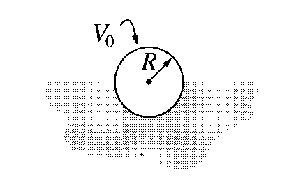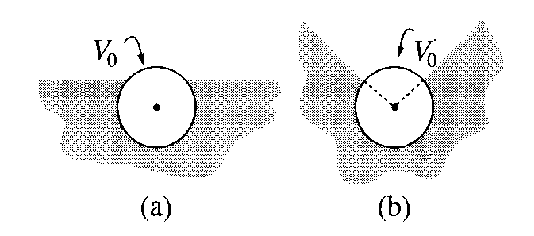Figure 1: A conducting sphere embedded in a dielectric in various ways.

2. A dielectric slab is partially inserted between between planar capacitor plates. Find the force acting on the slab of dielectric!
3. A small cavity is carved inside a large piece of dielectric material. Suppose that there is a uniform electric field,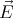0, in the dielectric. Find the electric field inside the cavity in the following cases:
1. The cavity is spherical.
2. A needle-shaped (long and thin) cavity, parallel to the field.
3. A wafer-shaped cavity, perpendicular to the field.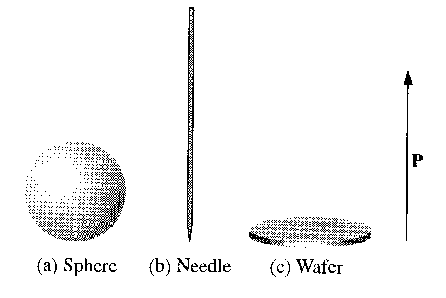(Problem 4.16 in Griffiths’s book.)

4. An emulsion is a mechanical mixture of two unblendable fluids. Consider an emulsion where droplets of a fluid of permittivity ε1 are dispersed in a fluid of permittivity ε0. The droplets amount to a fraction β of the total volume of the emulsion. Find the net permittivity of the emulsion!
5. We have a magnetic field whose magnitude is linearly varying in a direction perpendicular to the field lines: B = (B0 + kx)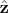.
1. Qualitatively describe the motion of a charged particle in this field.
2. Find an approximate formula for the drift velocity. Assume that in the region where the particle is moving |B - B0|≪ B0.

Back to the main page